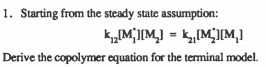# Starting from the steady state assumption: k, [M][M_) = \,[M]M, Derive the copolymer equation for the terminal model.

Question-AnswerCategory: ChemistryStarting from the steady state assumption: k, [M][M_) = \,[M]M, Derive the copolymer equation for the terminal model.

### Starting from the steady state assumption: k, [M][M_) = \,[M]M, Derive the copolymer equation for the terminal model.